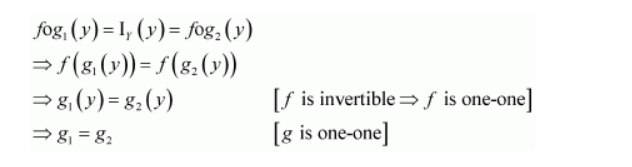# Let f: X → Y be an invertible function. Show that f has unique inverse.

Question:

Let fX → Y be an invertible function. Show that f has unique inverse.

(Hint: suppose g1 and g2 are two inverses of f. Then for all y ∈ Y,

fog1(y) = IY(y) = fog2(y). Use one-one ness of f).

Solution:

Let fX → Y be an invertible function.

Also, suppose $f$ has two inverses (say $g_{1}$ and $g_{2}$ ).

Then, for all $y \in Y$, we have:Hence, f has a unique inverse.